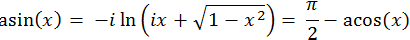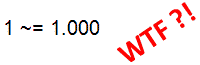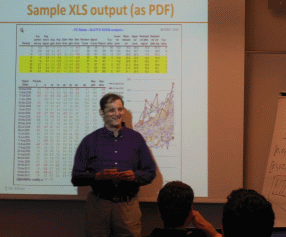# Matlab numerical gotchas

In deference to Halloween, I thought of posting a short note on a couple of Matlab numerical gotchas. They are not really undocumented – in some cases they are specifically documented and in other cases can be inferred from the documentation. What they share in common is that for some reason they are not widely known. Their importance comes from the fact that I often encounter them in Matlab code, and they often do not immediately cause syntax error, but rather more difficult-to-diagnose incorrect results. So I thought it would be worthwhile to formally document them here:

### 1. Inverse-trig of “invalid” values

This one came to me from Baruch Karlin:

In a program using math functions, it is easy to forget to limit the values of interim computations to the boundaries expected in the physical world. One specific example is that while we normally think of inverse trig functions (e.g., asin and acos) as returning the angles corresponding to the specified trig function result, in fact such inverse-trig functions are defined mathematically such that their range extends past the “standard” domain (-1 to +1 in the case of asin and acos):The result is that `asin(10)` is just as valid in Matlab as `asin(1)`. The results of such “invalid” values are standard complex values, which Matlab treats as regular numeric values. This is often an undesirable effect that would cause incorrect results downstream in the program code:

```>> value = some_computation(); >> result = asin(value) %value=10 in this example result = 1.5707963267949 - 2.99322284612638i```

As the snippet shows, it is sometimes difficult to immediately see that the `value` variable could receive an inappropriate value, resulting in a complex `result`. Assuming this is undesirable, we could clamp the value:

```value = some_computation(); value = min(max(real(value),-1),1); %value is clamped to [-1,+1] result = asin(value); % result is assured to be real```

### 2. Numeric (in)equalities

This is an oldie, in this case well predating Matlab, going back all the way to the early days of floating-point arithmetic. It is probably the most widely-known and yet most widely-encountered gotcha in any programming language since the days of yore. It is featured in the semi-official Matlab FAQ, which explains the reason that:```>> 1 == (3 -1.1 -0.9) ans = 0 %=false!   >> 2 -1.1 -0.9 ans = -1.11022302462516e-016```

The workaround is to use eps whenever comparison of non-integer numeric values is needed, and indirect comparison (e.g., < or >) rather than direct ones (== or ~=).

The reader in referred to the above-mentioned FAQ, or to one of the following resources:

### 3. Cube root returns a complex number

This one is also an oldie from the semi-official Matlab FAQ (a bit paraphrased):

Since 81/3=2, we naturally expect to find that (-8)1/3=-2. Unfortunately we find in Matlab that:

```>> (-8)^(1/3) ans = 1 + 1.73205080756888i```

In the same way there are two solutions (plus and minus) for the square root of a positive real number, there are multiple solutions for roots of negative (and complex) numbers. If you express a number as `A*eiθ`, its cube root takes the form (A1/3)*ei(θ+2kπ)/3, for k=0:2. Since -8 is 8e, θ=π or -π. Therefore, the cube roots of -8 are 2eiπ/3, 2e-iπ/3, and 2e. The last one simplifies to the expected real value of -2.

So we see that the cube root (and in general, the n-th root) always returns 3 roots (or in general, n roots), and Matlab chooses one of them, which may not necessarily be non-complex. As in the first gotcha, the resulting complex value is correct in the mathematical sense, but could well lead to erroneous results downstream in the Matlab code.

Matlab always returns the first solution counter-clockwise from the positive real axis. Armed with this knowledge, we can compute all or some particular root. For instance, if you want the negative real cube root, simply take the cube root of the absolute value of the number, and negate it. For a different wording and more information, see MathWorks Tech Solution 1-15M1N.

Joe Sababa suggested a method to find all the roots at once using roots on the polynomial, effectively solving `x3+8=0` for x:

```>> P=[1 0 0 8]; roots(P) % solve x^3 + 8 = 0 ans = -2 0.999999999999999 + 1.73205080756888i 0.999999999999999 - 1.73205080756888i```

(note the FP inaccuracy, discussed in the previous gotcha)

An alternate method to obtain -2 as a cube root of -8 is to use the nthroot function:

```>> x = nthroot(-8, 3) x = -2```

### 4. Usage of “+” and “-” in arrays

Usage of “+” and “-” operators is normally intuitive. Matlab knows when we refer to the unary operation and when to the binary one. But it gets somewhat less intuitive in numeric arrays:

```>> [1 -2 3 4 5] %unary minus ans = 1 -2 3 4 5   >> [1 - 2 3 4 5] % binary minus ans = -1 3 4 5     >> [1 +2 3 4 5] % unary plus ans = 1 2 3 4 5   >> [1 + 2 3 4 5] % binary plus ans = 3 3 4 5```

As can be seen, the extra space between the operator and the number caused Matlab to change its treatment of the operator. This also applies to programmatic values such as: `[value1 -value2 ...]`.Teaching how to customize Excel reports via Matlab (click for details)

A few months ago I presented a Matlab training course at some company. One of the attendees tried to programmatically modify an Excel worksheet in a Matlab program by directly accessing certain worksheet cells as ‘a3’, ‘b3’ etc. He used a simple loop such as this:

```for columnIdx = 1 : 5 cellAddress = ['a' +columnIdx-1 '3']; % should be, for example: 'c3' Excel.Range(cellAddress).Value = someValue; end```

Can you spot why Excel croaked when the Matlab program ran?

The workaround here is to either ensure that unary operators are directly adjacent to the values, or separate the values with a comma (or a semi-colon ; ) to prevent any misunderstanding by Matlab:

```>> [1, - 2, 3, 4, 5] ans = 1 -2 3 4 5   >> [1; - 2; 3; 4; 5] ans = 1 -2 3 4 5```

Do you have another similar gotcha that you’ve encountered? If so, please post a comment below.

(if there’s any interest, maybe I’ll follow up with some non-numeric gotchas for next year’s Halloween…)

Happy Halloween everyone!

Categories: Low risk of breaking in future versions, Stock Matlab function

Tags:Print

### 9 Responses to Matlab numerical gotchas

1.Walter Roberson on Facebook says:

Let A and B be positive. Then mod(-A,B) will return B itself, if A < eps(B), instead of returning something in [0,B) as would normally be expected for mathematical modulus operator.

2.Chandrakanth says:

I think I have come across quite such ‘gotachas’, but this is the only one i can recollect now.

```m>=3 % is fine. m>= 3 % notice the space before 3. This is fine as well. m >= 3 % notice space before 3 and after m. This too is fine. m >=3 % space only after m. This complains with an error.```
3.Wesley Brodsky says:

One problem I have noticed in the past, which might still exist, is one of the “creeping imaginary part”. After a long series of calculations with all real numbers, a teeny-weenie imaginary part (<10^-10 times real part) appears. This causes an error if input to a function that only accepts real numbers. This is not the same as the cube-root issue, since the real part is what I expect. I place x = real(x) at random places in the calculations to avoid this. The problem might no longer exist, but I have not experimented to find out.

Has anyone else seen this?

4.the cyclist says:

I find it surprising that min(0,NaN) returns a result of 0 rather than NaN.

There is some discussion of this here:

5.Guillaume says:

Hmm, 2e^2iπ/3 is not a cube root of -8. The correct formula for nth roots of Ae^iθ is A^1/n * e^i(θ/n + 2kπ/n). Hence, cube roots of -8 are 2e^iπ (k=1), 2e^-iπ (k=2) and 2e^iπ/3 (k=3).

•Yair Altman says:

@Guillaume – thanks for the correction. It serves me right for copying wiki math without double checking. I have now corrected both the main article and the referenced FAQ one.

BTW – it appears that like me, you have also gotten an error in your comment: I believe that the correct roots are 2e^iπ, 2e^iπ/3 and 2e^-iπ/3, and not as you have stated.6.Daniel E. Shub says:

Despite the documentation for the colon function I cannot help but expect x = 0:0.1:1; to be equal to [0*0.1, 1*0.1, 2*0.1, …, 10*0.1] as that is how I describe the function working. This can cause a gotcha when checking for subsets

>> x = 0:0.1:1;
>> y = 0:0.1:0.5;
>> isequal(x(1:6), y(1:6))

7.Malcolm Lidierth says:

Take a Java method with signatures accepting either:

a scalar int
a double[]

as input.

MATLAB’s Java reflection mechanism will pass a scalar flint as a double[] to the second method by default. To invoke the method with an int input, it needs to be cast explicitly to an int.

8.Sami Varjo says:

It was “cool” to note that the weak typing favors integers in arrays. One would expect that the more weak variable would be promoted as the floating point presentation is usually default, however:

```>> myUint8Var = uint8(0); >> newVector = [myUint8Var 510.14 45.24 1.2] newVector = 0 255 45 1```

This actually happens also if you preallocate an array for a number of vectors where you assign the values:

```>> array = zeros(3,4,'double'); >> array(1,:) = [myUint8Var 510.14 45.24 1.2] array = 0 255 45 1 0 0 0 0 0 0 0 0```

So also here explicit type cast for myUint8Var is required for expected assumed behavior (R2012a). This one haunted me quite a while…